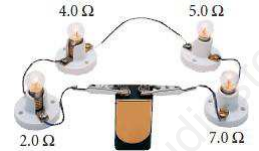# CBSE Class 10 Physics Tungsten Assignment

Read and download free pdf of CBSE Class 10 Physics Tungsten Assignment. Get printable school Assignments for Class 10 Physics. Standard 10 students should practise questions and answers given here for Tungsten Physics in Grade 10 which will help them to strengthen their understanding of all important topics. Students should also download free pdf of Printable Worksheets for Class 10 Physics prepared as per the latest books and syllabus issued by NCERT, CBSE, KVS and do problems daily to score better marks in tests and examinations

## Assignment for Class 10 Physics Tungsten

Class 10 Physics students should refer to the following printable assignment in Pdf for Tungsten in standard 10. This test paper with questions and answers for Grade 10 Physics will be very useful for exams and help you to score good marks

### Tungsten Class 10 Physics Assignment

1. Why tungsten is used exclusively for filaments of electric bulbs?

2. When you break a bar magnet in half, how many poles does each piece have?

3. Which of the compass-needle orientations in the figure below might correctly describe the magnet’s field at that point?4. A 9.0 V battery is connected to four light bulbs, as shown at right. Find the equivalent resistance for the circuit and the current in the circuit.5. A series combination of two resistors, 7.25 Ω and 4.03 Ω, is connected to a 9.00 V battery.

a. Calculate the equivalent resistance of the circuit and the current.

b. What is the potential difference across each resistor?

6. A length of wire is cut into five equal pieces. The five pieces are then connected in parallel, with the resulting resistance being 2.00 Ω. What was the resistance of the original length of wire before it was cut up?

7. Write any three applications of heating effect of electric current?

8. Express 1kWh in SI unit.

9. A 400 W refrigerator operates for 16h per day. Calculate the cost to operate it for 30 days at Rs 3.40 per kWh.

Please click the below link to access CBSE Class 10 Physics Tungsten Assignment